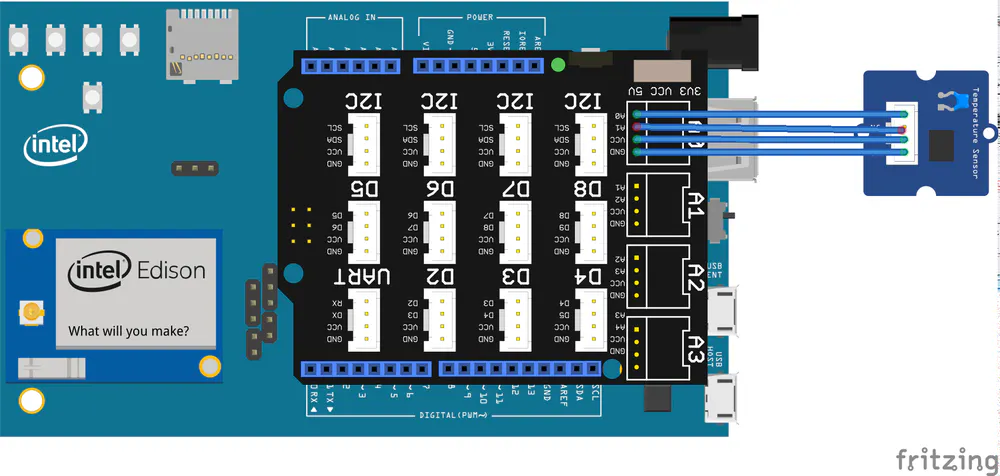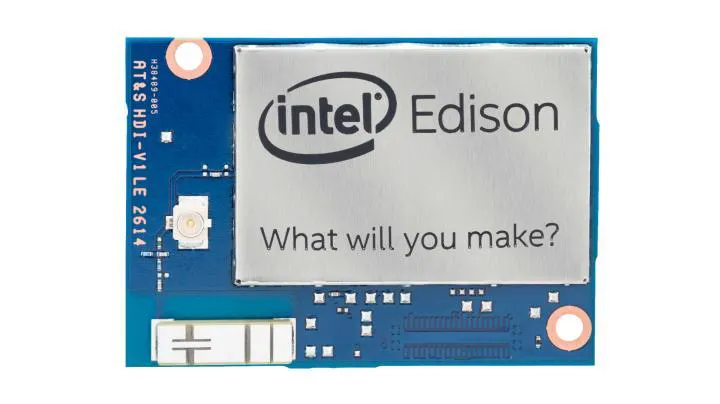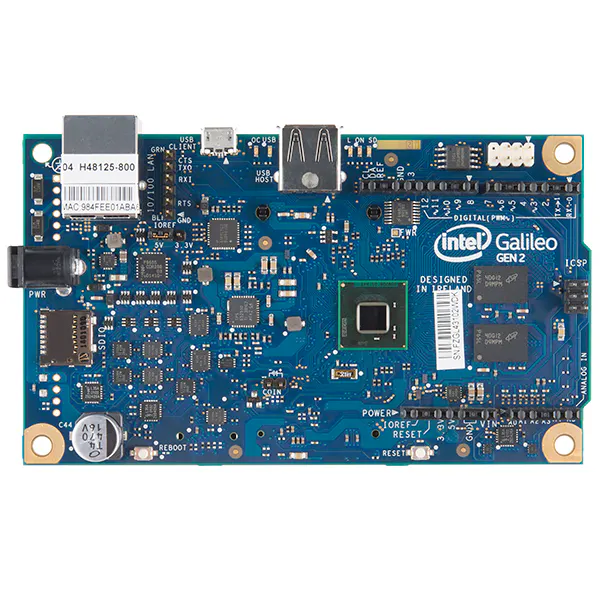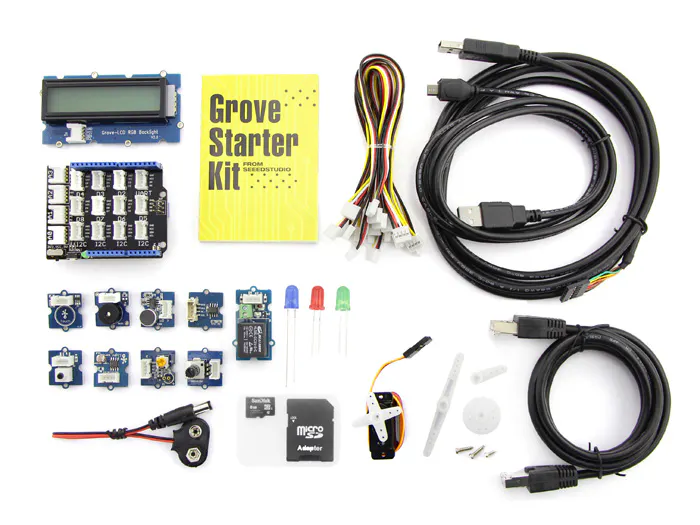# C/C++ on Intel Edison/Galileo – part5:Temperature sensor

In this part, we will interface a temperature sensor to Galileo/Edison

BeginnerFull instructions provided30 minutes3## Things used in this project

### Hardware componentsIntel Edison
×1Intel Galileo Gen 2
×1Seeed Grove starter kit plus for Intel Edison
×1

## Code

### Code snippet #1

Plain text
```#include <stdio.h>
#include <mraa.h>
#include <math.h>           // for the log function

const int B  = 4275;         // B value for thermistor
const int R0 = 100;          // R0 is 100k ohms

int main(void)
{
mraa_aio_context   adcPin;           // used as AIO context
float              R, temperature;   // Used in calculations

/* Step1: Initialize the mraa */
mraa_init();

/* Step2: Initlaize the ADC pin */

/* Step4: Apply the transformation */
R = 1023.0/((float)adcValue)-1.0;    // Promote to float
R = 100000.0*R;

temperature = 1.0/(log(R/100000.0)/B+1/298.15)-273.15;

/* Step5: Print the temperature */
printf ("The temperature is %0.2f degree Celcius\n", temperature);

return 0;
}
```

### Code snippet #4

Plain text
```R = 1023.0/((float)adcValue)-1.0;    // Promote to float
R = 100000.0*R;
temperature = 1.0/(log(R/100000.0)/B+1/298.15)-273.15;

printf ("The temperature is %0.2f degree Celcius\n", temperature);
```

### Code snippet #7

Plain text
```#include <stdio.h>  // for printf()

/* Step1: Bring in the upm */
#include <upm/grove.h>

int main(void)
{
int temperature;

/* Step2: Instantiate an instance of Grove temperature sensor */

/* Step3: Record the temperature and print */
temperature = temp->value();
printf ("The temperature is %d degree Celcius\n", temperature);

return 0;
}
```